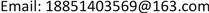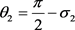﻿ 镇江市产业结构与碳排放耦合协调发展分析 Analysis on the Coordinated Development of Industrial Structure and Carbon Emission in Zhenjiang City

Sustainable Development
Vol. 09  No. 03 ( 2019 ), Article ID: 31270 , 9 pages
10.12677/SD.2019.93050

Analysis on the Coordinated Development of Industrial Structure and Carbon Emission in Zhenjiang City

Zhirong Liu, Na Lu, Yuehan Qiu, Xinmiao Wu, Kai Zhou

School of Finance and Economics, Jiangsu University, Zhenjiang JiangsuReceived: June 23rd, 2019; accepted: July 8th, 2019; published: July 15th, 2019ABSTRACT

In the context of low-carbon economy development, this paper takes Zhenjiang City, a low-carbon pilot city in China, as the research object. Firstly, it comprehensively measures carbon emission levels from four aspects: per capita carbon emissions, carbon emission density, carbon emission intensity and carbon productivity. The high-level, rationality and concentration of industrial structure in Zhenjiang City were calculated. Then, the carbon emission level of Zhenjiang City and the advanced degree, rationality and concentration of industrial structure were analyzed and coordinated. The conclusions are as follows: 1) The carbon emission level and its various indicators are significantly different. The order of carbon emission from high to low is the municipal district, Jurong City, Danyang City and Yangzhong City. 2) The distribution of industrial structure has also shown great differences among different regions. The three levels are relatively high in Danyang City, followed by Yangzhong City, Municipal District and Jurong City. 3) The overall coupling coordination degree of Zhenjiang City is low, the carbon emission level and the industrial structure evolution are not coordinated, and the space presents a large imbalance. Finally, this paper proposes policy recommendations on how Zhenjiang City can achieve high-quality coupling and coordinated development of industrial structure adjustment and carbon emissions.

Keywords:Industrial Structure, Carbon Emissions, Coupling CoordinationCopyright © 2019 by authors and Hans Publishers Inc.1. 引言

2. 研究方法与数据来源

2.1. 研究方法

2.1.1. 碳排放综合指数Table 1. Carbon emission indicators and their implications

${P}_{\theta ij}={x}_{\theta ij}/{\sum }_{\theta =1}^{r}{x}_{\theta ij}$ (1)

${e}_{j}=-\frac{1}{\mathrm{ln}r}{P}_{\theta ij}\mathrm{ln}{P}_{\theta ij}$ (2)

${W}_{j}=\left(1-{e}_{j}\right)/{\sum }_{j=1}^{n}\left(1-{e}_{j}\right)$ (3)

${x}_{\theta ij}^{*}=\frac{{x}_{\theta ij}-\mathrm{min}\left({x}_{ij}\right)}{\mathrm{max}\left({x}_{ij}\right)-\mathrm{min}\left({x}_{ij}\right)}$ (4)

${x}_{\theta ij}^{*}=\frac{\mathrm{max}\left({x}_{ij}\right)-{x}_{\theta ij}}{\mathrm{max}\left({x}_{ij}\right)-\mathrm{min}\left({x}_{ij}\right)}$ (5)

${E}_{\theta i}={\sum }_{j=1}^{n}\left({W}_{j}\cdot {x}_{\theta ij}^{*}\right)$ (6)

2.1.2. 产业结构的分解描述

$IH={\theta }_{1}+{\theta }_{2}$

${\theta }_{1}=\pi -{\mu }_{2}-{\mu }_{3}$$\theta =\mathrm{arccos}\left[\frac{{\sum }_{i=1}^{n}\left({x}_{i}{x}_{i},0\right)}{{\left({\sum }_{i=1}^{n}{x}_{i}^{2}\right)}^{\frac{1}{2}}{\left({\sum }_{i=1}^{n}{x}_{i,0}^{2}\right)}^{\frac{1}{2}}}\right]$$\left(n=2,3\right)$ (7)

$TL={\sum }_{i=1}^{n}\left(\frac{{Y}_{i}}{Y}\right)\mathrm{ln}\left(\frac{{Y}_{i}}{{L}_{i}}/\frac{Y}{L}\right)$ (8)

$I=\left(A-R\right)/\left(M-R\right)$ (9)

2.1.3. 耦合协调评价模型

$C={\left\{\frac{E\left(x\right){S}_{i}\left(y\right)}{{\left[\frac{E\left(x\right)+{S}_{i}\left(y\right)}{2}\right]}^{2}}\right\}}^{k}$ (10)

$R=\sqrt{C×\left(0.6E\left(x\right)+0.4{S}_{i}\left(y\right)\right)}$ (11)Table 2. The reference standard of coupling & coordinating stage

2.2. 变量选取及数据来源

3. 结果分析

3.1. 碳排放水平结果分析Table 3. Carbon emission index and carbon emission level in Jiangsu Province and Zhenjiang City from 2006-2016

3.2. 产业结构解构及空间分布

1) 产业结构高级度方面。丹阳市高级度指数为0.67，属于产业结构高级化发达地区；句容市的高级度指数为0.58，远远低于全市平均水平0.63，属于产业结构高级化落后地区；扬中市、市辖区的高级度指数都在0.64左右，属于产业结构高级化发展中地区。以上结果说明丹阳市在2006年以来产业结构重心由第一产业向二、三产业转移的速度最快，这与丹阳市近些年来大力发展二、三产业有着密切的关系。

2) 产业结构集中度方面。市辖区、扬中市的集中度指数分别为0.42、0.39，属于产业结构高集中度地区；句容市的集中度指数为0.18，远低于全市平均水平0.23，属于产业结构低集中度地区；丹阳市集中度指数为0.25，属于中等集中度地区。以上结果的出现是由于市辖区第三产业产值有着较大的比重，扬中市重工业集聚，而句容市与丹阳市各产业的发展比较均衡。

3) 产业结构合理度方面。丹阳市、扬中市合理度指数分别为0.12、0.14，属于产业结构高合理度地区；市辖区、句容市的合理度指数分别为0.34、0.31，远高于全市平均水平0.23，属于产业结构低合理度地区，产业结构与均衡状态的偏差程度很大，见表4，与产业结构合理化之间还用很长的路要走。Table 4. Industrial structure analysis in Jiangsu Province and Zhenjiang City from 2006 to 2016

3.3. 碳排放与产业结构耦合协调度分析

1) 耦合协调度时间变化分析

2) 耦合协调度空间变化分析

3) 从耦合协调度分布来看，碳排放水平与产业结构高级度方面，市辖区处于低协调耦合阶段，句容市处于高协调耦合阶段，丹阳市、扬中市处于极协调耦合阶段。碳排放水平与产业结构合理度方面，市辖区、丹阳市、扬中市处于低协调耦合阶段，句容市处于高协调耦合阶段。碳排放水平与产业结构集中度方面，市辖区与句容市处于低协调耦合阶段，丹阳市处于中协调耦合阶段，扬中市处于高协调耦合阶段。

4) 2006~2016年间，镇江市各地区碳排放水平和产业结构耦合协调度与地区经济发展水平具有不对称性。人均GDP最高的市辖区的碳排放水平和产业结构高级度、合理度、集中度的耦合协调度均为低协调分离阶段，人均GDP最低的句容市的碳排放水平和产业结构高级度、合理度、集中度的耦合协调度分别处于高协调磨合阶段、高协调磨合阶段与低协调分离阶段，见表5，导致这种现象产生的原因有很多，其中最主要的是由于各地区三次产业的发展力度不同。此外，地区间经济发展、资源禀赋及人口密度都存在差异，同时，不同地区经济发展过程中政策取向存在差异，也是导致产业结构耦合协调度与地区经济发展水平具有不对称性的原因。Table 5. The coupling degrees function and coupling coordination degrees between Carbon emission level and industrial structure in Jiangsu Province and Zhenjiang City from 2006 to 2016

4. 结论与讨论

4.1. 结论

1) 从碳排放水平的空间分布和区域差异看，镇江市碳排放水平优于江苏省整体；镇江市各地区人均碳排放量、碳排放密度、碳排放强度、碳生产力差异比较明显，各项指标中，基本上都是扬中市位居首位，丹阳市和句容市交替处于二、三位，而市辖区则均相对较低。在镇江市各地区中，市辖区应注意提高碳生产力，扬中市、丹阳市、句容市应在人均碳排放量、碳排放密度、碳排放强度方面三管齐下，优化碳排放水平。

2) 一般而言，在研究产业结构3个维度空间分布和发展态势方面，产业结构高级度和合理度分布空间结构应该比较相似，而产业结构集中度的分布与前两者差异较大，但在对镇江市进行产业结构高级度、合理度和集中度进行分析时发现并未出现上述现象。究其主要原因是镇江市近年来各地区产业结构调整较快，且在调整的过程中未注意在地区和产业之间的协调相互发展。

3) 从碳排放水平与产业结构的耦合协调关系看，镇江市各地区整体耦合协调度偏低，碳排放水平与产业结构演化没有协调发展，且空间呈现很大的不均衡态势。产业结构高级度与碳排放水平耦合度较高，说明产业结构高级化是改善碳排放水平的有效途径。产业结构合理度与集中度则与碳排放水平的耦合度较低。在碳排放水平与产业结构偏离最严重的地区是市辖区，其产业发展的高度分散会严重阻碍其经济的发展。

4.2. 讨论

Analysis on the Coordinated Development of Industrial Structure and Carbon Emission in Zhenjiang City[J]. 可持续发展, 2019, 09(03): 412-420. https://doi.org/10.12677/SD.2019.93050

1. 1. 谢晓晶. 中国碳排放与产业结构关联研究[D]: [硕士学位论文]. 北京: 北方工业大学, 2013.

2. 2. 刘凤朝, 孙玉涛. 技术创新、产业结构调整对能源消费影响的实证分析[J]. 中国人口•资源与环境, 2008, 18(3): 108-113.

3. 3. 王修华, 王翔. 产业结构升级与低碳经济发展的耦合研究[J]. 软科学, 2012, 26(3): 29-32.

4. 4. 王洋帅, 童纪新. 江苏省产业结构升级、城市化与碳排放的动态关系——基于VAR模型的实证研究[J]. 河南科学, 2016, 34(5): 798-804.

5. 5. 徐胜, 司登奎. 结构转型、能源效率对低碳经济的异质性影响——基于省际数据的面板协整分析[J]. 软科学, 2014, 28(7): 6-10+39.

6. 6. 江洪, 赵宝福. 碳排放约束下能源效率与产业结构解构、空间分布及耦合分析[J]. 资源科学, 2015, 37(1): 152-162.

7. 7. 郑少智, 陈志辉. 产业结构高级化与经济增长关系实证研究——基于全国、广东省及广州市数据的对比分析[J]. 产经评论, 2011(3): 55-60.

8. 8. 干春晖, 郑若谷, 余典范. 中国产业结构变迁对经济增长和波动的影响[J]. 经济研究, 2011, 46(5): 4-16+31.

9. 9. 关伟, 许淑婷. 辽宁省能源效率与产业结构的空间特征及耦合关系[J]. 地理学报, 2014, 69(4): 520-530.

10. 10. 马丽, 金凤君, 刘毅. 中国经济与环境污染耦合度格局及工业结构解析[J]. 地理学报, 2012, 67(10): 1299-1307.

11. 11. 单豪杰. 中国资本存量K的再估算: 1952-2006年[J]. 数量经济技术经济研究, 2008, 25(10): 17-31.